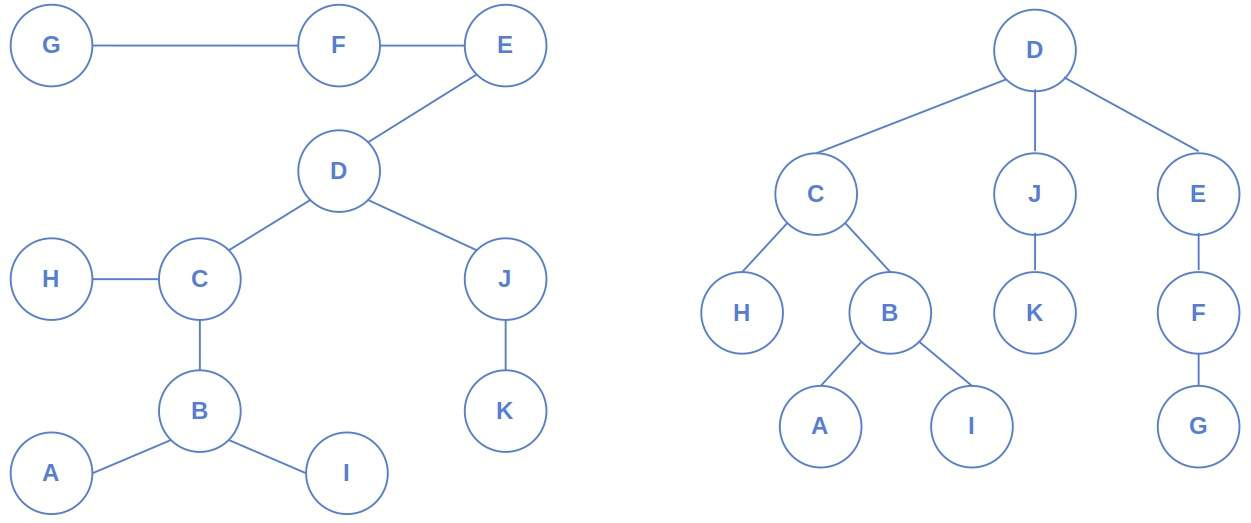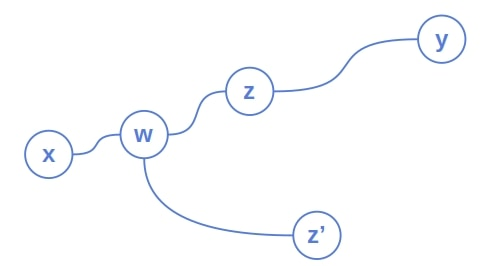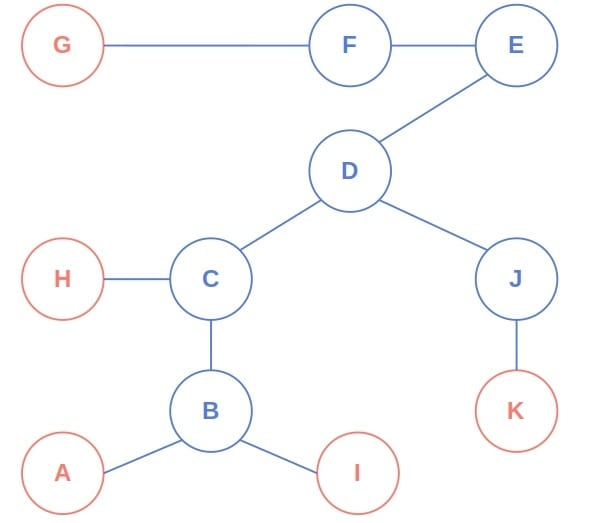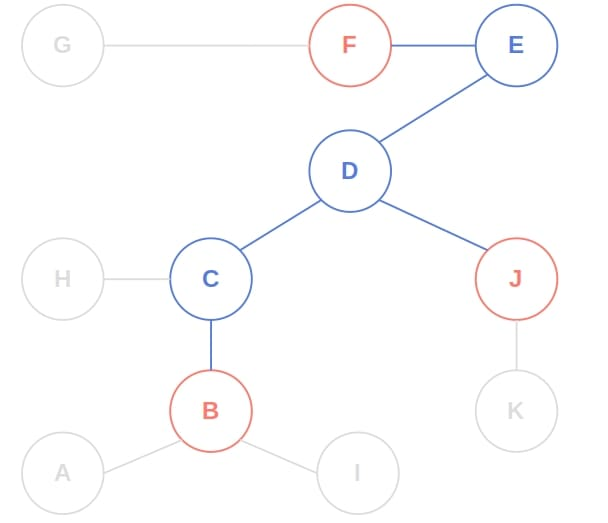## 1. Introduction

In this tutorial, we’ll show how to find the central nodes of a tree.

## 2. Tree Centers

The graph theory defines a tree center as the node with minimal eccentricity (the length of the longest path to any other node):On the left, we have an undirected and unrooted tree. On the right, we see the same tree rooted at its center.

That’s why we care about tree centers. Sometimes, we are given a tree as a regular graph, so no node is marked as the root. Our job is to root the tree, and doing so at the central node pays off in many applications.

We’ll assume that the trees we’re working with are unweighted, undirected, and unrooted.

### 2.1. Center and Diameter

The diameters of a tree are the longest paths in it. As we saw, there can be more than one. We can prove that the center of a tree must lie in the middle of its diameter(s).

Letandbe the endpoints of a diameter containing an odd number of nodes, and letbe its middle node.

Let another nodebe the tree’s center andthe diameter node closest to:Depending on whetheris betweenandorand, either the pathis longer thanorthan. That’s impossible since, by our assumption,has the minimum eccentricity. So,can’t be the center, which means thatmust be.

If the diameter contains an even number of nodes, there are two middle nodes, and both will be the tree’s centers.

## 3. Algorithm

That gives us an idea of how to find the center(s) in unweighted trees.

Since central nodes are in the middle of a diameter, we can start by removing the endpoint nodes one layer at a time until we reach the middle nodes.

Each node in the outmost layer has only one neighbor, which we can call its parent. If we remove the surface nodes, their parents will have only one neighbor. As we move toward the center, the eccentricity decreases by one since all the edges have the same cost (1). So, we repeat this process by moving inward until we get one or two central nodes:

``````

Although we assume the tree is unrooted, this algorithm implicitly defines its structure. The nodes it processes in the same iteration belong to the same level. Those processed in iterationare the parents of the nodes handled in iteration, and the tree’s center becomes its root.

### 3.1. Example

Let’s run an example. In the first iteration, the surface nodes are,,,, and:In the second one, those are,, and:We processandin the third iteration and leave the loop withas the found center.

### 3.2. Correctness

Letbe the tree we get by removing theth iteration’s surface layer from. Those trees are implicit: we don’t construct them but can derive them during the algorithm’s execution.

Our loop invariant is thathas the same center nodes as the input tree. Let’s prove it by induction.

The base case is, i.e., the situation before the main loop, which trivially holds:has the same centers as itself.

Let’s assume that the invariant is true at the end of theth iteration. Does it hold at the end of the iteration? In other words, doandhave the same centers?

We can show that’s the case by noting that a diameter’s endpoints have the degree one. If we remove such nodes from, all the diameters will shrink by a node from both sides. So, those in the middle will stay the same. As a result,andhave the same centers.

Consequently, the nodes we end up with are‘s central nodes. There can be two centers at most because each diameter has either one or two middle nodes. Furthermore, we’ve shown that the diameters intersect at the centers!

### 3.3. Complexity

No node in an-ary tree has more thanneighbors. In the worst case, a node’s edges are in a linked list, so we need to traverse it to find its degree. In such a tree withnodes, computing the degrees takestime. Ifis a constant, that will reduce to. Similarly, if we store neighbors in an array, we can read its length intime, which gives the overall complexity of.

Further, each edge is processed in the main loop only once. Since a tree withnodes hasedges, the loop’s time complexity is.

So, this algorithm runs in linear time.

## 4. Conclusion

In this article, we showed how to find an unweighted tree’s central nodes in linear time. They are in the middle of the longest path(s), and there are at most two centers in each tree.